Hits: 4

# Deletion from a B-tree

#### In this tutorial, you will learn how to delete a key from a b-tree. Also, you will find working examples of deleting keys from a B-tree in C, C++, Java and Python.

Deleting an element on a B-tree consists of three main events: searching the node where the key to be deleted exists, deleting the key and balancing the tree if required.

While deleting a tree, a condition called underflow may occur. Underflow occurs when a node contains less than the minimum number of keys it should hold.

The terms to be understood before studying deletion operation are:

1. Inorder Predecessor
The largest key on the left child of a node is called its inorder predecessor.
2. Inorder Successor
The smallest key on the right child of a node is called its inorder successor.

## Deletion Operation

Before going through the steps below, one must know these facts about a B tree of degree m.

1. A node can have a maximum of m children. (i.e. 3)
2. A node can contain a maximum of `m - 1` keys. (i.e. 2)
3. A node should have a minimum of `⌈m/2⌉` children. (i.e. 2)
4. A node (except root node) should contain a minimum of `⌈m/2⌉ - 1` keys. (i.e. 1)

There are three main cases for deletion operation in a B tree.

### Case I

The key to be deleted lies in the leaf. There are two cases for it.

1. The deletion of the key does not violate the property of the minimum number of keys a node should hold.In the tree below, deleting 32 does not violate the above properties.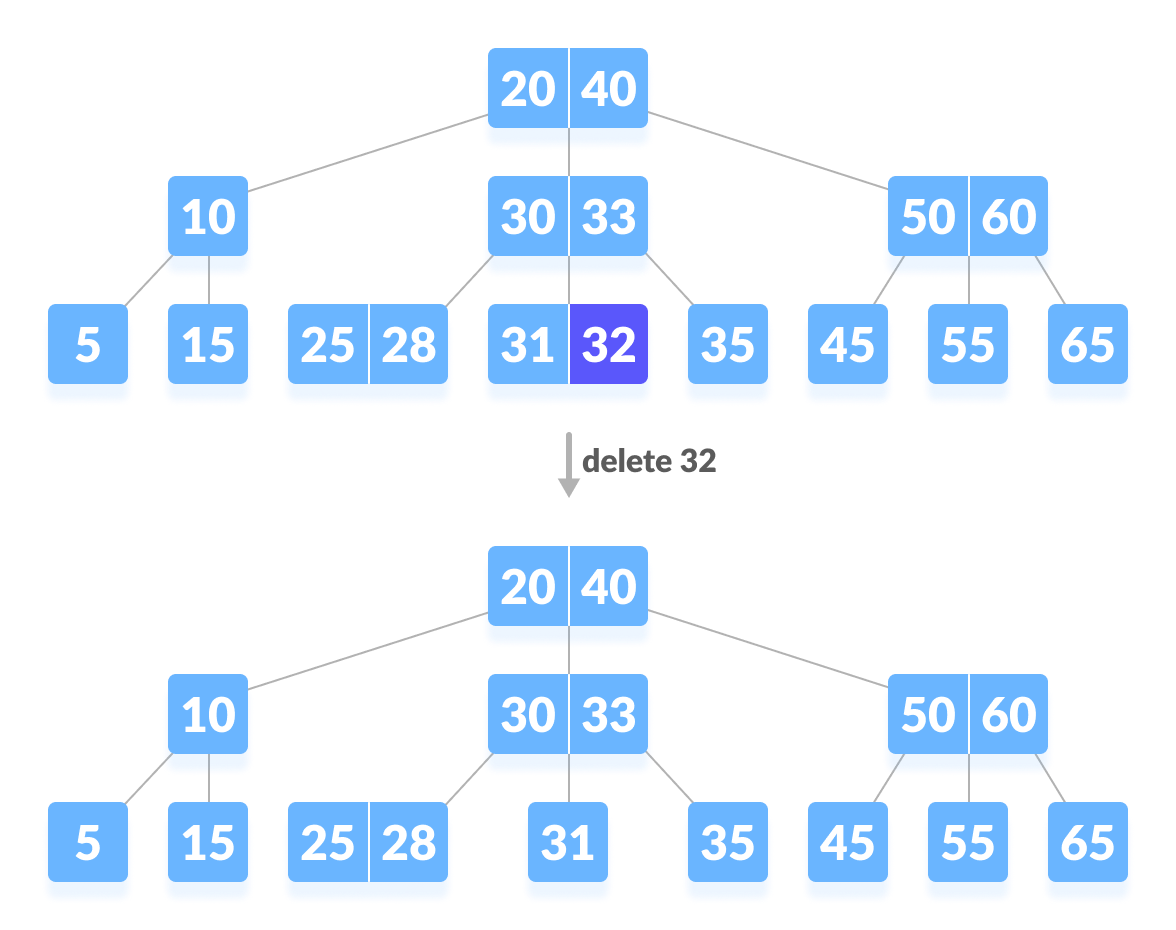Deleting a leaf key (32) from B-tree
2. The deletion of the key violates the property of the minimum number of keys a node should hold. In this case, we borrow a key from its immediate neighboring sibling node in the order of left to right.First, visit the immediate left sibling. If the left sibling node has more than a minimum number of keys, then borrow a key from this node.

Else, check to borrow from the immediate right sibling node.

In the tree below, deleting 31 results in the above condition. Let us borrow a key from the left sibling node.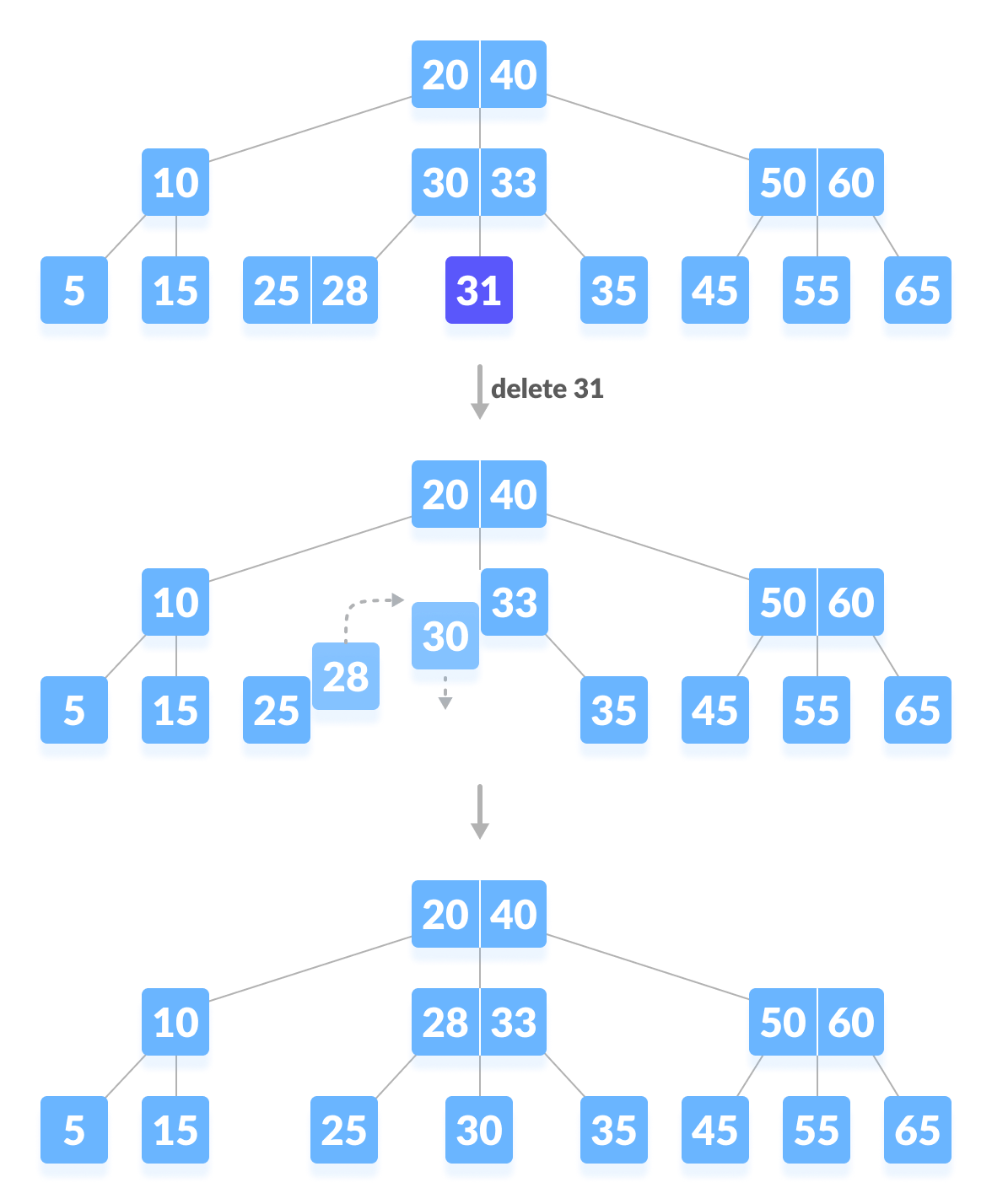Deleting a leaf key (31)

If both the immediate sibling nodes already have a minimum number of keys, then merge the node with either the left sibling node or the right sibling node. This merging is done through the parent node.

Deleting 30 results in the above case.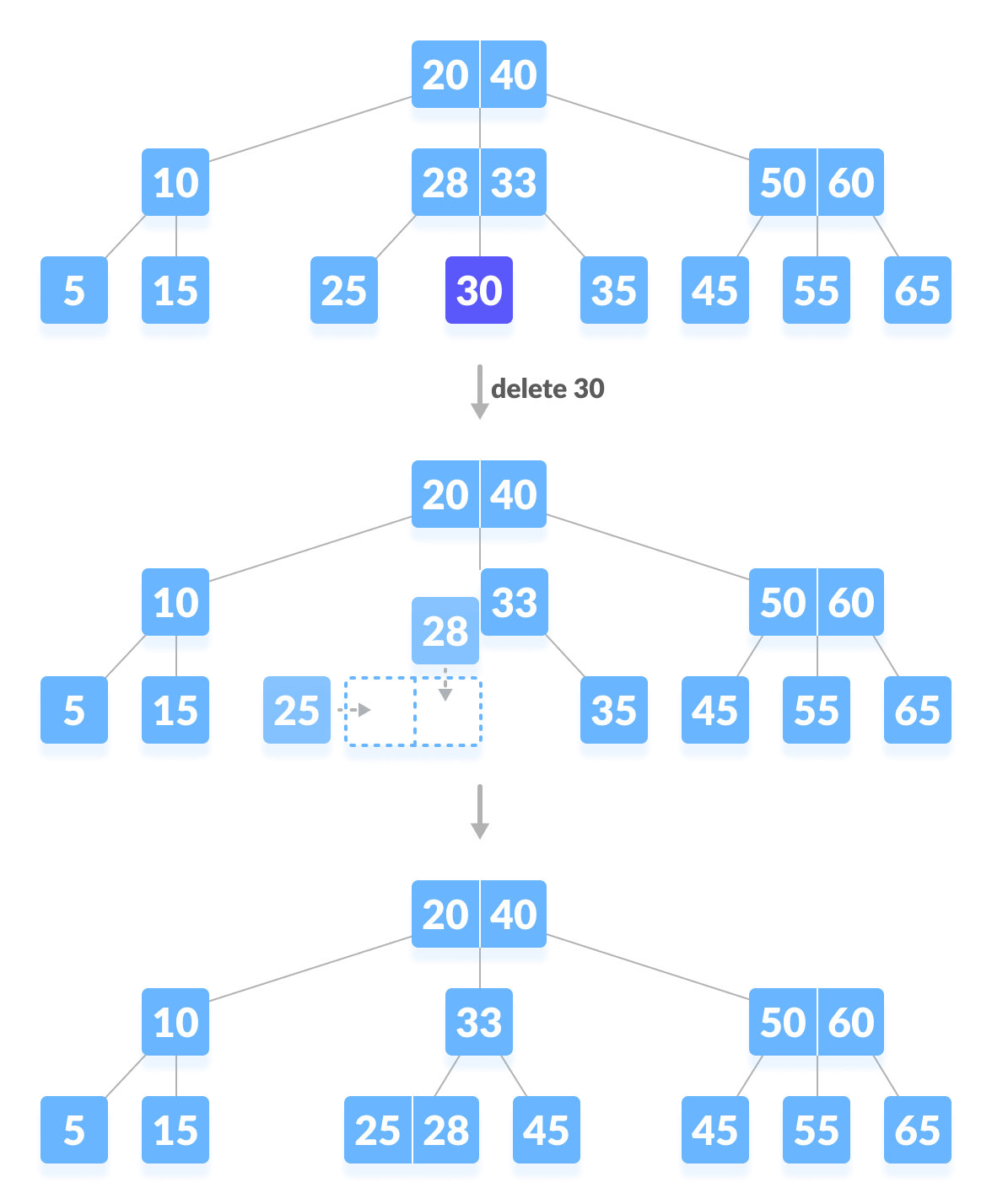Delete a leaf key (30)

### Case II

If the key to be deleted lies in the internal node, the following cases occur.

1. The internal node, which is deleted, is replaced by an inorder predecessor if the left child has more than the minimum number of keys.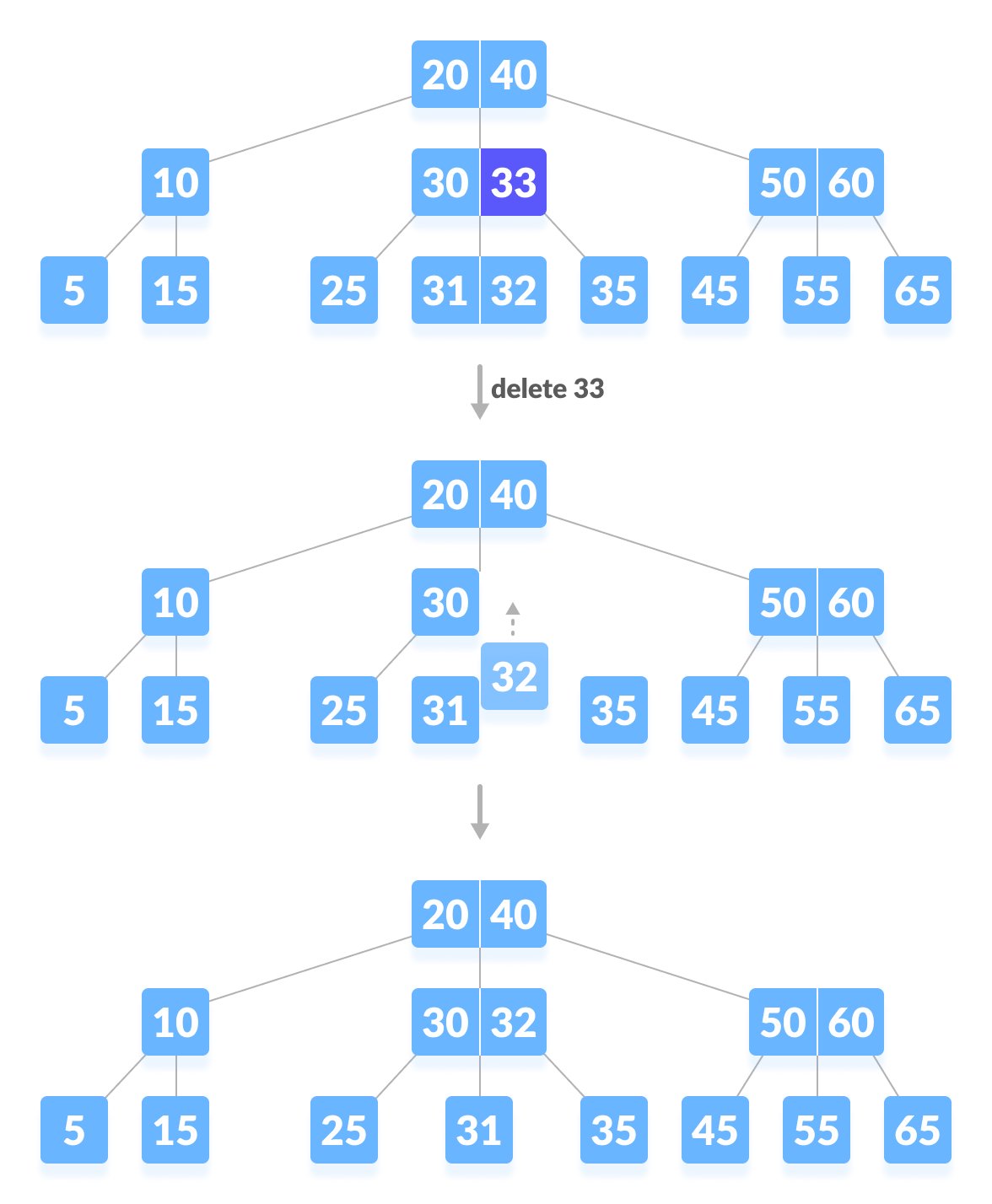Deleting an internal node (33)
2. The internal node, which is deleted, is replaced by an inorder successor if the right child has more than the minimum number of keys.
3. If either child has exactly a minimum number of keys then, merge the left and the right children.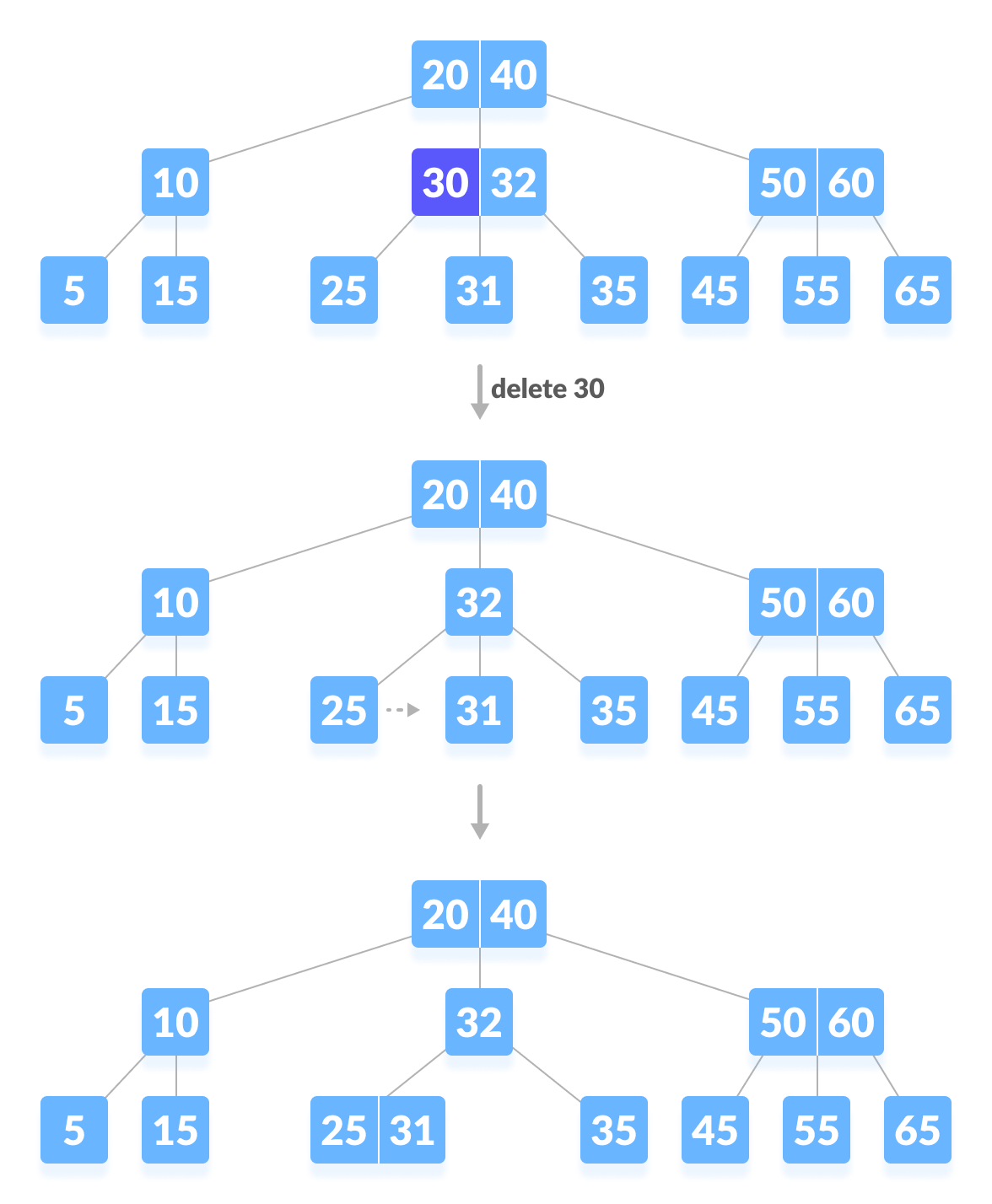Deleting an internal node (30)

After merging if the parent node has less than the minimum number of keys then, look for the siblings as in Case I.

### Case III

In this case, the height of the tree shrinks. If the target key lies in an internal node, and the deletion of the key leads to a fewer number of keys in the node (i.e. less than the minimum required), then look for the inorder predecessor and the inorder successor. If both the children contain a minimum number of keys then, borrowing cannot take place. This leads to Case II(3) i.e. merging the children.

Again, look for the sibling to borrow a key. But, if the sibling also has only a minimum number of keys then, merge the node with the sibling along with the parent. Arrange the children accordingly (increasing order).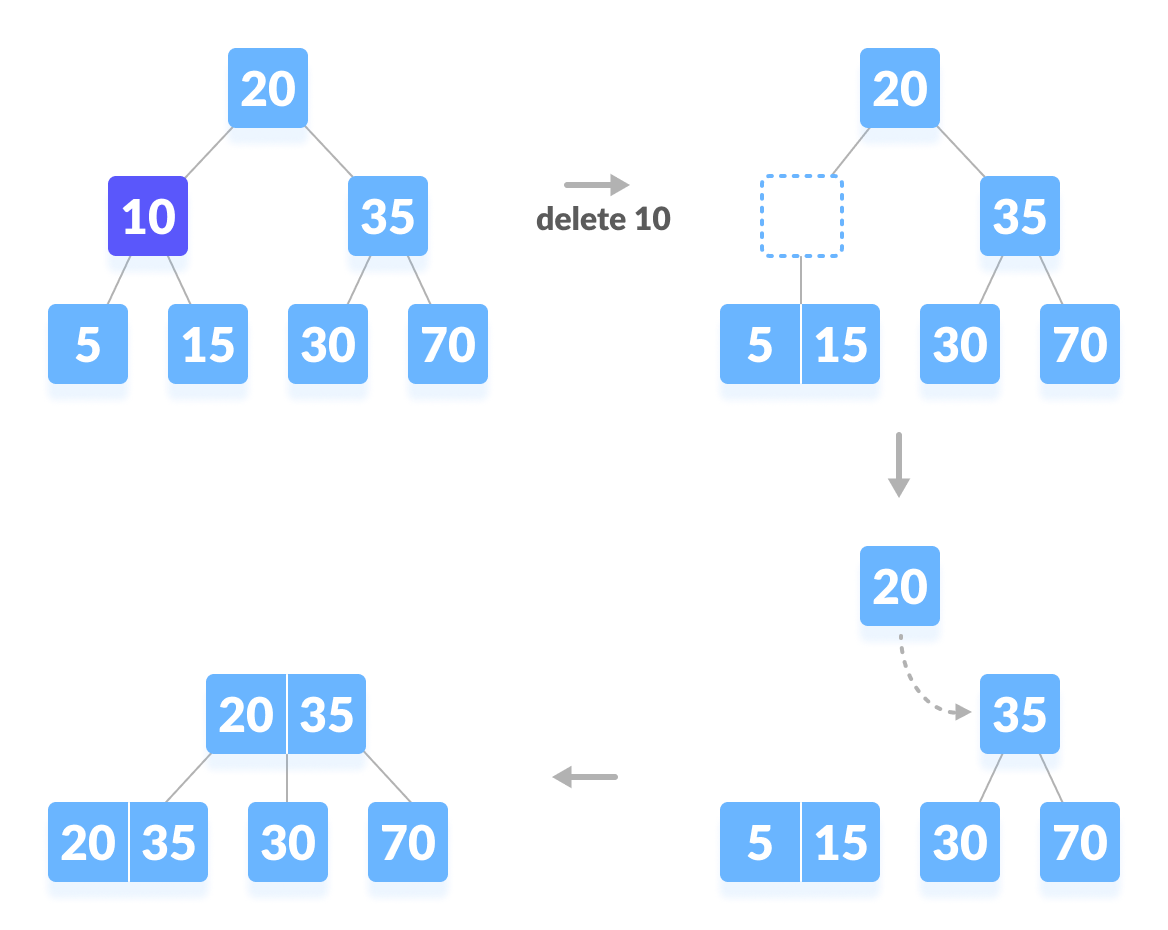Deleting an internal node (10)

## Python Examples

``````/* Deleting a key on a B-tree in Python */

/* Btree node */
class BTreeNode:
def __init__(self, leaf=False):
self.leaf = leaf
self.keys = []
self.child = []

class BTree:
def __init__(self, t):
self.root = BTreeNode(True)
self.t = t

/* Insert a key */
def insert(self, k):
root = self.root
if len(root.keys) == (2 * self.t) - 1:
temp = BTreeNode()
self.root = temp
temp.child.insert(0, root)
self.split_child(temp, 0)
self.insert_non_full(temp, k)
else:
self.insert_non_full(root, k)

/* Insert non full */
def insert_non_full(self, x, k):
i = len(x.keys) - 1
if x.leaf:
x.keys.append((None, None))
while i >= 0 and k < x.keys[i]:
x.keys[i + 1] = x.keys[i]
i -= 1
x.keys[i + 1] = k
else:
while i >= 0 and k < x.keys[i]:
i -= 1
i += 1
if len(x.child[i].keys) == (2 * self.t) - 1:
self.split_child(x, i)
if k > x.keys[i]:
i += 1
self.insert_non_full(x.child[i], k)

/* Split the child */
def split_child(self, x, i):
t = self.t
y = x.child[i]
z = BTreeNode(y.leaf)
x.child.insert(i + 1, z)
x.keys.insert(i, y.keys[t - 1])
z.keys = y.keys[t: (2 * t) - 1]
y.keys = y.keys[0: t - 1]
if not y.leaf:
z.child = y.child[t: 2 * t]
y.child = y.child[0: t - 1]

/* Delete a node */
def delete(self, x, k):
t = self.t
i = 0
while i < len(x.keys) and k > x.keys[i]:
i += 1
if x.leaf:
if i < len(x.keys) and x.keys[i] == k:
x.keys.pop(i)
return
return

if i < len(x.keys) and x.keys[i] == k:
return self.delete_internal_node(x, k, i)
elif len(x.child[i].keys) >= t:
self.delete(x.child[i], k)
else:
if i != 0 and i + 2 < len(x.child):
if len(x.child[i - 1].keys) >= t:
self.delete_sibling(x, i, i - 1)
elif len(x.child[i + 1].keys) >= t:
self.delete_sibling(x, i, i + 1)
else:
self.delete_merge(x, i, i + 1)
elif i == 0:
if len(x.child[i + 1].keys) >= t:
self.delete_sibling(x, i, i + 1)
else:
self.delete_merge(x, i, i + 1)
elif i + 1 == len(x.child):
if len(x.child[i - 1].keys) >= t:
self.delete_sibling(x, i, i - 1)
else:
self.delete_merge(x, i, i - 1)
self.delete(x.child[i], k)

/* Delete internal node */
def delete_internal_node(self, x, k, i):
t = self.t
if x.leaf:
if x.keys[i] == k:
x.keys.pop(i)
return
return

if len(x.child[i].keys) >= t:
x.keys[i] = self.delete_predecessor(x.child[i])
return
elif len(x.child[i + 1].keys) >= t:
x.keys[i] = self.delete_successor(x.child[i + 1])
return
else:
self.delete_merge(x, i, i + 1)
self.delete_internal_node(x.child[i], k, self.t - 1)

/* Delete the predecessor */
def delete_predecessor(self, x):
if x.leaf:
return x.pop()
n = len(x.keys) - 1
if len(x.child[n].keys) >= self.t:
self.delete_sibling(x, n + 1, n)
else:
self.delete_merge(x, n, n + 1)
self.delete_predecessor(x.child[n])

/* Delete the successor */
def delete_successor(self, x):
if x.leaf:
return x.keys.pop(0)
if len(x.child.keys) >= self.t:
self.delete_sibling(x, 0, 1)
else:
self.delete_merge(x, 0, 1)
self.delete_successor(x.child)

/* Delete resolution */
def delete_merge(self, x, i, j):
cnode = x.child[i]

if j > i:
rsnode = x.child[j]
cnode.keys.append(x.keys[i])
for k in range(len(rsnode.keys)):
cnode.keys.append(rsnode.keys[k])
if len(rsnode.child) > 0:
cnode.child.append(rsnode.child[k])
if len(rsnode.child) > 0:
cnode.child.append(rsnode.child.pop())
new = cnode
x.keys.pop(i)
x.child.pop(j)
else:
lsnode = x.child[j]
lsnode.keys.append(x.keys[j])
for i in range(len(cnode.keys)):
lsnode.keys.append(cnode.keys[i])
if len(lsnode.child) > 0:
lsnode.child.append(cnode.child[i])
if len(lsnode.child) > 0:
lsnode.child.append(cnode.child.pop())
new = lsnode
x.keys.pop(j)
x.child.pop(i)

if x == self.root and len(x.keys) == 0:
self.root = new

/* Delete the sibling */
def delete_sibling(self, x, i, j):
cnode = x.child[i]
if i < j:
rsnode = x.child[j]
cnode.keys.append(x.keys[i])
x.keys[i] = rsnode.keys
if len(rsnode.child) > 0:
cnode.child.append(rsnode.child)
rsnode.child.pop(0)
rsnode.keys.pop(0)
else:
lsnode = x.child[j]
cnode.keys.insert(0, x.keys[i - 1])
x.keys[i - 1] = lsnode.keys.pop()
if len(lsnode.child) > 0:
cnode.child.insert(0, lsnode.child.pop())

/* Print the tree */
def print_tree(self, x, l=0):
print("Level ", l, " ", len(x.keys), end=":")
for i in x.keys:
print(i, end=" ")
print()
l += 1
if len(x.child) > 0:
for i in x.child:
self.print_tree(i, l)

B = BTree(3)

for i in range(10):
B.insert((i, 2 * i))

B.print_tree(B.root)

B.delete(B.root, (8,))
print("n")
B.print_tree(B.root)``````

## Deletion Complexity

Best case Time complexity: `Θ(log n)`

Average case Space complexity: `Θ(n)`

Worst case Space complexity: `Θ(n)`

# Special 95% discount

## 2000+ Applied Machine Learning & Data Science Recipes

### Portfolio Projects for Aspiring Data Scientists: Tabular Text & Image Data Analytics as well as Time Series Forecasting in Python & R## Two Machine Learning Fields

There are two sides to machine learning:

• Practical Machine Learning:This is about querying databases, cleaning data, writing scripts to transform data and gluing algorithm and libraries together and writing custom code to squeeze reliable answers from data to satisfy difficult and ill defined questions. It’s the mess of reality.
• Theoretical Machine Learning: This is about math and abstraction and idealized scenarios and limits and beauty and informing what is possible. It is a whole lot neater and cleaner and removed from the mess of reality.

Data Science Resources: Data Science Recipes and Applied Machine Learning Recipes

Introduction to Applied Machine Learning & Data Science for Beginners, Business Analysts, Students, Researchers and Freelancers with Python & R Codes @ Western Australian Center for Applied Machine Learning & Data Science (WACAMLDS) !!!

Latest end-to-end Learn by Coding Recipes in Project-Based Learning:

Applied Statistics with R for Beginners and Business Professionals

Data Science and Machine Learning Projects in Python: Tabular Data Analytics

Data Science and Machine Learning Projects in R: Tabular Data Analytics

Python Machine Learning & Data Science Recipes: Learn by Coding

R Machine Learning & Data Science Recipes: Learn by Coding

Comparing Different Machine Learning Algorithms in Python for Classification (FREE)

`Disclaimer: The information and code presented within this recipe/tutorial is only for educational and coaching purposes for beginners and developers. Anyone can practice and apply the recipe/tutorial presented here, but the reader is taking full responsibility for his/her actions. The author (content curator) of this recipe (code / program) has made every effort to ensure the accuracy of the information was correct at time of publication. The author (content curator) does not assume and hereby disclaims any liability to any party for any loss, damage, or disruption caused by errors or omissions, whether such errors or omissions result from accident, negligence, or any other cause. The information presented here could also be found in public knowledge domains.  `